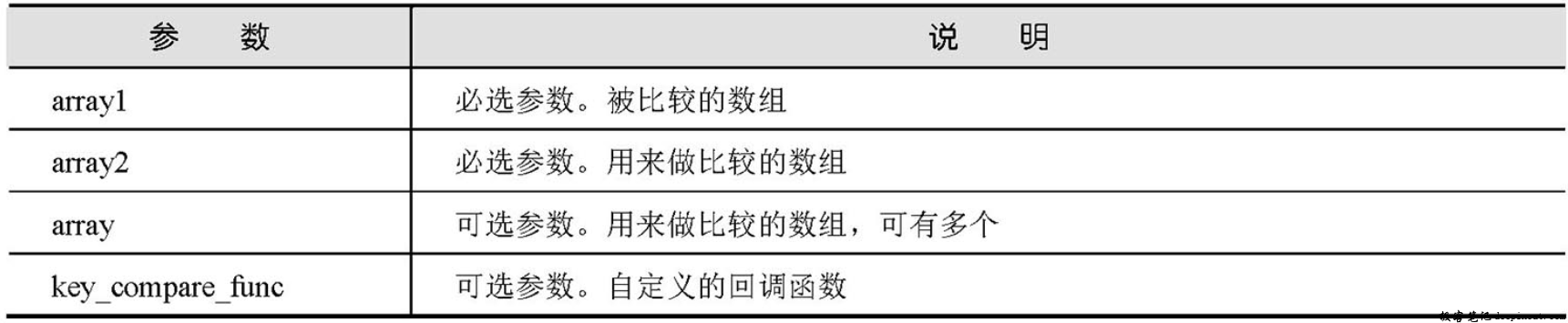# PHP array_diff_uassoc函数

PHP array_diff_uassoc函数——用回调函数做索引检查来计算数组的差集，array_diff_uassoc函数可用用户提供的回调函数做索引检查来计算数组的差集。返回一个数组，该数组包括了所有在被比较数组中，但是不在任何其他参数数组中的值。键名也可以用于比较。array_diff_uassoc()函数仅检查多维数组中的一维。该函数与array_diff_assoc()函数的行为正好相反，后者是用内部函数进行比较的。

## PHP array_diff_uassoc函数 语法

array array_diff_uassoc ( array array1, array array2 [,array...,callback key_compare_func])


array_diff_uassoc()函数的参数说明如下表所示。## PHP array_diff_uassoc函数 示例

<?php
function key_compare_func($a,$b){
if ($a ===$b) {                      //用if 语句判断参数$a 和参数$b 是否相等
return 0;                          //如果相等则返回0
}
return ($a >$b)? 1:-1;               //如果参数$a 大于$b，返回整数1
}
$array1 = array("asp" => "极客笔记", "php" => "deepinout", "java" => "深入浅出"); //声明数组$array1
$array2 = array("asp" => "极客笔记", "深入浅出", "deepinout"); //声明数组$array2
$result = array_diff_uassoc($array1, $array2, "key_compare_func"); //应用array_diff_uassoc()函数 print_r($result);                        //输出计算数组的差集
?>


Array
(
[php] => deepinout
[java] => 深入浅出
)


• 回顶